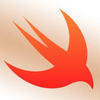PRO

# Keep on failing with the challenge.

Let's add some code to the body of the function. Get rid of the existing return statement that's in there and in this task we're going to write out code to take a Celcius temperature value passed in and convert it to Fahrenheit.

To convert from Celcius to Fahrenheit - multiply the value by 9, divide the resulting value by 5 and then add 32.

Once you have the Fahrenheit value, return it from the function.

functions.swift
```// Enter your code below

func temperatureInFahrenheit(temperature: Double) -> Double {

}
```MOD

Hi there,

So, you have the passed in value called `temperature`. You want to do the specified mathematics on that and return the result.

Take `temperature`, & multiply it by 9:

```temperature * 9
```

divide the result by 5:

```(temperature * 9) / 5
```

```((temperature * 9) / 5) + 32
```

And return that from the function:

```return ((temperature * 9) / 5) + 32
```

Make sense?

Steve.I am not sure you were you are failing but I am assuming on task 2. For task 2 you need to return the operation that is going to give you the Fahrenheit value. In task 3 you are just calling the function and passing in a value

```func temperatureInFahrenheit(temperature: Double) -> Double {

return temperature * 9 /  5 + 32

}

let fahrenheitTemp = temperatureInFahrenheit(temperature: 24.0)
```